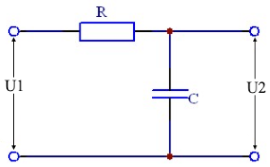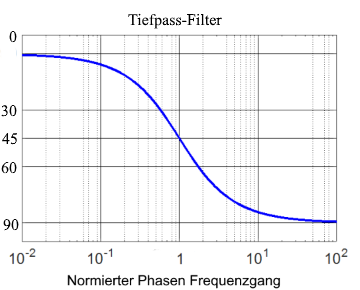# RC low pass filter

Calculator and formulas for calculating the parameters of an RC low pass

## RC low pass online calculator

This function calculates the properties of a low-pass filter consisting of a resistor and a capacitor. The output voltage, attenuation and phase rotation are calculated for the given frequency.

RC low pass calculator

 Input Resistor mΩ Ω kΩ MΩ Capacitor F mF µF nF pF Frequency Hz kHz MHz GHz Input voltage µV mV V kV Decimal places 0 1 2 3 4 6 8 10 Result Reactance XC Output voltage U2 Attenuation dB Phase shift φ Cutoff frequency Impedance Z Voltage UR Current I Current I Time constant τ$$\displaystyle C$$ = Capacity [F]

$$\displaystyle R$$ = Resistance [Ω]

$$\displaystyle U_1$$ = Input voltage [V]

$$\displaystyle U_2$$ = Output voltage [V]

$$\displaystyle X_C$$ = Capacitive reactance [Ω]

$$\displaystyle φ$$ = Phase angle [°]

$$\displaystyle Z$$ = Input impedance [Ω]

$$\displaystyle I$$ = Current [A]

$$\displaystyle U_R$$ = Voltage at the restistor [V]

## Formulas for the RC low pass filter

### Calculate the voltage ratio

The output voltage U2 of an RC low pass is calculated according to the following formula.

$$\displaystyle U_2=U_1 ·\frac{1} {\sqrt{1 + (2 · π · f · R · C)^2}}$$

or easier if XC is known

$$\displaystyle U_2=U_1 ·\frac{X_C}{\sqrt{R^2 + X_C^2}}$$
$$\displaystyle X_C=\frac{1}{2 π · f ·C}$$

### Attenuation in decibels

At the resonance frequency, the damping is 3 dB. If the input and output voltage are known, the attenuation for all frequencies can easily be calculated using the following formula.

$$\displaystyle V_u=20 · lg \left(\frac{U_2}{U_1} \right)$$

If the voltages are not known, the following formula is used.

$$\displaystyle V_u=20·lg\left(\frac{1} {\sqrt{1 + (2 · π · f · R · C)^2}}\right)$$

or simply shown

$$\displaystyle V_u=20·lg\left(\frac{1} {\sqrt{1 + (ω · R · C)^2}}\right)$$

### Phase shift

In an RC low pass, the output voltage lags the input voltage by 0 ° - 90 °, depending on the frequency. At the resonance frequency, the phase shift is -45 °. At low frequencies, it tends to 0. At high frequencies, the phase shift in the direction of -90 °. The phase shift can be calculated using the following formula.

$$\displaystyle φ=acos \left(\frac{U_2}{U_1} \right))$$
$$\displaystyle φ= arctan (ω · R ·C)$$### Cutoff frequency

At the limit frequency fg bzw. ωg the value of the amplitude-frequency response (ie the magnitude of the transfer function) is 0.707. This corresponds to -3 dB.

$$\displaystyle 0.707= \frac{1}{\sqrt{2}}$$

#### Cutoff frequency formulas

$$\displaystyle ω_g= \frac{1}{R ·C} ⇒$$
$$\displaystyle f_g=\frac{1}{2·π·R·C}$$

$$\displaystyle R=\frac{1}{2·π·f_g·C}$$
$$\displaystyle C=\frac{1}{2·π·f_g·R}$$

#### Impedance

$$\displaystyle Z=\sqrt{X_C^2 + R^2}$$

#### Current

$$\displaystyle I=\frac{U}{Z}$$

#### Restistor voltage

$$\displaystyle U_R=R ·I$$

#### Time constant

$$\displaystyle τ=C ·R$$